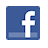Games
Problems
Go Pro!

# Factor Me

Pro Problems > Math > Algebra > Expressions > Simplifying and Factoring

## Factor Me

Factor the following expression over the set of reals:

a3b + 7ab - 9a3bx2 - 63abx2

Extra: Factor over the set of complex numbers.

Presentation mode
Problem by Mr. Twitchell

## Solution

In order to make it feasible for teachers to use these problems in their classwork, no solutions are publicly visible, so students cannot simply look up the answers. If you would like to view the solutions to these problems, you must have a Virtual Classroom subscription.Assign this problem
Click here to assign this problem to your students.

## Similar Problems

### Fifth Degree Factoring

Factor the following completely:

x5 - 2x4 - 5x3y2 + 10x2y2 + 4xy4 - 8y4

### Ugly Simplification

Simplify the following expression completely, assuming that x>0.x6
· x-1 + 33
x4
·3
x-1
+ 2
x1/2 · x1/3 · x1/6 + 1

### Factor Me

Factor the following expression completely:

-3x3 + 4x2 + 4x - y2x + 4yx2

### Factoring with M and N

Factor the following quadratic expression in N and M:

M2 + 4N2 + 5M + 10N + 4MN + 4

### Special K

For some constant k, the following division has no remainder. Find the value of k.

(x3 + kx2 - 14x - 12) ÷ (x - 3).

### Two Variable Factoring

Factor the following completely:

(a4b5)3
a2b
-
11(a3b4)2
ab
+ 28

### Binomial Factoring

Factor 3x7y + 96x2y11

### Factoring with X, Y, and Z

Factor the following expression:

x2 + 4y2 + 9z2 + 4xy + 6xz + 12yz.

# Featured Games on This Site

Match color, font, and letter in this strategy game
Trap all the dots in this problem-solving puzzle

# Blogs on This SiteReviews and book lists - books we love!The site administrator fields questions from visitors.Like us on Facebook to get updates about new resources